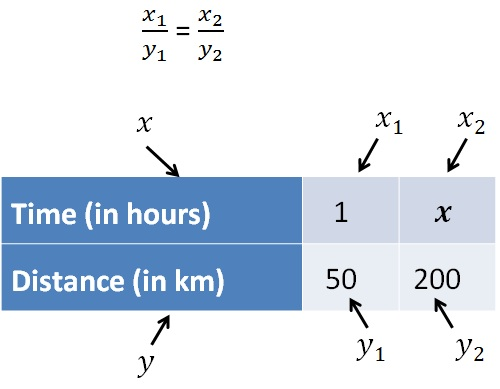Direct Proportion

Chapter 11 Class 8 Direct and Inverse Proportions
Concept wise

When one quantity increases, the other quantity also increases

Like,

If distance increases, time taken also increases

If number of books purchased increases, total cost also increases

So, we can say that

Distance and Time are directly proportional

Similarly,

Number of books purchased and cost are directly proportional

#### How to solve questions with Direct Proportion?

Let’s take an example

Suppose it takes us 1 hour to drive 50 km. How many hours would it take to drive 200 km?

Given that,

It takes 1 hour to drive 50 km

We need to find how  many hours would it take to drive 200 km

Let the time taken to drive 200 km be x hours

Now, we draw a table

 Time (in hours) 1 x Distance (in km) 50 200

Now, as distance increases,

time taken will also increase

So, Time and Distance are in direct proportion

So, we write numbers asSo,

x 1 /  y 1  = x 2 /y 2

1/50=x/200

1/50  × 200 = x

1/5  × 20 = x

4 = x

x = 4

So, it takes 4 hours to travel 200 km

Let’s take another example

Suppose we have brought 10 books for Rs 1000. How much is the price of 45 books?

Given that,

Cost of 10 books is Rs 1000

We need to find price of 45 books

Let cost of 45 books be Rs y

Now, we draw a table

 Time (in hours) 10 45 Distance (in km) 1000 y

Now, as number of books increases,

cost of books will also increase

So, they are in direct proportion

So, we write numbers as

x 1 /y 1  = x /y 2

10/1000=45/y

10 × y =   45 × 1000

y = 1/10 × 45 × 1000

y = 45 × 100

y = 4500

So, 45 books will cost Rs 4500

Learn in your speed, with individual attention - Teachoo Maths 1-on-1 Class